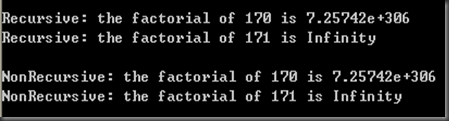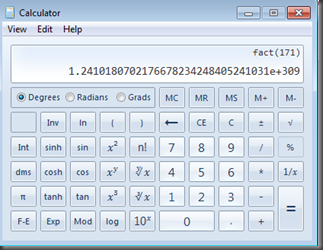# Too Big to Be Accurate(1): Which is the Most Powerful Calculator in the World?

Calculate the factorial of 171 (171!)? Just TRY! It is equal to 171*170*169*….2*1.

### 1. Google calculator

As Google fanatics, I first try to search the answer via Google:Whoops, nothing interested returned! Type “170!” and get the output:Why kinda things happened in this calculator? 171! is just equal to 171*170!.

### 2. Excel

Switch to Excel spreadsheet. Function fact(*) used:

### 3. SAS

Google and Excel may be the niche players in calculators’ family. Why not try to use some programming languages?

As a SAS programmer, my handy tool is SAS of course.

First, I use SAS data step with its build-in function fact(*):

data null;
x=fact(170);
y=fact(171);
put x= y=;
run;

and I get

NOTE: Invalid argument to function FACT at line 49 column 7.
x=7.257416E306 y=.
x=7.257416E306 y=. ERROR=1 N=1
NOTE: Mathematical operations could not be performed at the following places. The results of the operations have been set to missing values.

Expected or unexpected? I don’t know how this fact(*) function is defined, and  try to define a function to calculate the factorials by myself. In SAS 9.2, you can use PROC FCMP(also available at 9.1.3 as a experimental procedure):

proc fcmp outlib = work.funcs.math ;
function factorial(k) ;
if k = 0 then return(1) ;
z = k ; *preserve k ;
x = factorial(k-1) ;
k = z ; *recover k ;
k = k * x ;
return(k) ;
endsub ;
quit ;

options cmplib=work.funcs ;

Use this self-defined function to get 170!

proc fcmp ;
x = factorial (170) ;
put x = ;
run ;

The FCMP procedure returns

x=7.257416E306

Try to calculate 171! ?

proc fcmp ;
y = factorial (171) ;
put y = ;
run ;

Just get the overflow error. The interaction stops at 170!:

ERROR: An overflow occurred during execution in function ‘factorial’ in statement number 7 at   line 10 column 1.
The statement was:
1      (10:1)    k = (k=171) * (x=7.257416E306)

The above function definitions use recursion. Recursion may have some limitation on efficiency. We could try the loop without recursion. SAS/IML doesn’t support recursion. Let SAS/IML to the court:

proc iml;
start factorial (n);
fact=1;
do i=1 to n;
fact=fact*i;
end;
return (fact);
finish factorial;

x= factorial (170);
print x;

y= factorial (171);
print y;
quit;

Again, I get 170!

x
7.257E306

and a overflow error for 171!

y= factorial (171);
ERROR: Overflow error in *.

Turing, Von Neumann and Tony, what happened?

### 4. R

When SAS failed, lots of voices pop up: use R! OK, Rction!

> x=factorial(170);x
 7.257416e+306
> y=factorial(171);y
Warning message:
In factorial(171) : value out of range in ‘gammafn’
 Inf

### 5. C++

I don’t want to lose my patience. Think C++(use both recursive and non-recursive methods):

#include
using namespace std;

double factRecursive(double num);
double factNonRecursive(double num);

int main()
{
cout<    cout<<“Recursive: the factorial of 170 is “<    cout<<“Recursive: the factorial of 171 is “<    cout<

cout<<“NonRecursive: the factorial of 170 is “<    cout<<“NonRecursive: the factorial of 171 is “<    cout<

return 0;
}

double factRecursive (double num)
{
if (num==0)
return 1;
else
return num*factRecursive(num-1);
}

double factNonRecursive (double num)
{
double fact=1;
for (double i=2;i<=num;i++) fact *=i;
return fact;
}

Unfortunately, same story once more:Well. The story’s played out like this. It may be not the limitable of the language but the machine. I check which is the largest numbers my computer supports:

#include
#include

using namespace std;

int main()
{
cout<<“maxinum double value of machine: “<  return 0;
}

`maxinum double value of machine: 1.79769e+308`

Now everything’s in the open. The factorial of 170 is about 7.257416e+306. 171! is too big to be supported by my PC.

(Note: I put these codes in http://codepad.org, a online complier. if you don’t have any C++ complier in your machine, you can see the codes and outputs in:http://codepad.org/xnneavsw  and http://codepad.org/3FeEC9t2)

### 6. WolframAlpha

Struggled for hours, I turn to WolframAlpha computing platform. It returns the factorial of 171 AT LAST:

### 7. Windows Calculator

I try to use Windows build-in calculator. Amazing, it is powerful:### 8. Python

Return to programming language.  First, I defined a function(recursive version) in Python and then use its MATH library:

>>> def factorial(n):

if n==0:

return 1

else:

return n*factorial(n-1)

>>> factorial(170)

7257415615307998967396728211129263114716991681296451376

5435777989005618434017061578523507492426174595114909912

3783852077666602256544275302532890077320751090240043028

0058295603966612599658257104398558294257568966313439612

2625710949468067112055688804571933402126614528000000000

00000000000000000000000000000000L

>>> factorial(171)

1241018070217667823424840524103103992616605577501693185

3889518036119960752216917529927519781204875855764649595

0167038705280988985869071076733124203221848436431047357

7889968548278290754541561964852153468318044293239598173

6968996572359039476161522785581800611763651084288000000

00000000000000000000000000000000000L

>>> import math

>>> math.factorial(171)

``````<p>
1241018070217667823424840524103103992616605577501693185
</p>

<p>
3889518036119960752216917529927519781204875855764649595
</p>

<p>
0167038705280988985869071076733124203221848436431047357
</p>

<p>
7889968548278290754541561964852153468318044293239598173
</p>

<p>
6968996572359039476161522785581800611763651084288000000
</p>

<p>
00000000000000000000000000000000000L
</p>
``````

Amazing, Python beats up C++!

(to be continued :

Too Big to Be Accurate(2): Approximation

)